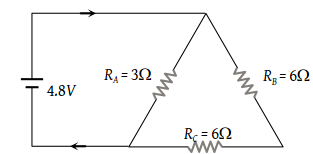# NEET Questions Solved

PMT - 1999

The current in the given circuit is(1) 8.31 A

(2) 6.82 A

(3) 4.92 A

(4) 2 A

(4) 6Ω and 6Ω are in series, so effective resistance is 12Ω which is in parallel with 3Ω, so

$\frac{1}{R}=\frac{1}{3}+\frac{1}{12}=\frac{15}{36}$$R=\frac{36}{15}$

$I=\frac{V}{R}=\frac{4.8×15}{36}=2\text{\hspace{0.17em}}A$

Difficulty Level:

• 8%
• 9%
• 14%
• 71%
Crack NEET with Online Course - Free Trial (Offer Valid Till August 23, 2019)